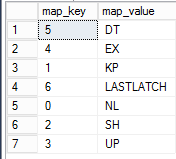# How to identify contention in TempDb

TempDb database is used to store all temp tables created for our applications. Whether explicit or implicit created, all temp tables activity is done in tempdb, creating a bottleneck.

For this reason, it’s important to identify tempdb contention. Tempdb contention will appear in the form of latches. Latch is a synchronization object that protects internal memory structures. In some ways they are similar to locks, but for internal memory structures. We need to identify latches in tempdb and avoid them.

There are two kinds of tempdb contention:

Allocation page contention, that happens when multiple sessions try to access allocation pages in the data file, like PFS, GAM and SGAM pages. The solution for this kind of contention is to add more data files to tempdb, so the access will be spread through the multiple files, eliminating the contention.

System table contention, that happens when multiple sessions try to access the tempdb system tables. Add more files doesn’t solve this kind of contention, the only solution is to change the code to avoid create temp tables so often.

We can use extended events to identify contention in tempdb. SQL Server has the event latch_suspend_end we can use. The event latch_supend_begin happens when a session try to acquire a latch and is blocked, so the session will wait until it can get the latch and latch_suspend_end will then occurs.

We need to filter the session to capture only events in tempdb with duration greater than zero. We can also filter the latch mode (SH, UP, EX). To find the correct values to use in latch mode filter we need to use the following query:

```SELECT map_key,
map_value
FROM   sys.dm_xe_map_values
WHERE  NAME = N'latch_mode'
```The XE session will become like this:

```CREATE event session [tempdb] ON server ADD event sqlserver.latch_suspend_end( WHERE (
[package0].[equal_uint64]([database_id],(2))
AND
(
[package0].[equal_uint64]([mode],(4))
OR
[package0].[equal_uint64]([mode],(2))
OR
[package0].[equal_uint64]([mode],(3))
)
AND
[package0].[greater_than_uint64]([duration],(0))
)
go
```

After creating and running the XE session, we need to retrieve the tempdb latch information and group the information to identify the amount of latches we have in tempdb. The best approach is to create a function to retrieve the latch information and group the resulting information from the function.

The function will be the following:

```CREATE FUNCTION [dbo].[LerTempContention]
(
-- Add the parameters for the function here
@xel varchar(500),
@xem varchar(500)
)
RETURNS TABLE
AS
RETURN
(
with qry as (
select
theNodes.event_data.value('(data[@name="database_id"]/value)','bigint') as database_id,
theNodes.event_data.value('(data[@name="class"]/value)','varchar(50)') as class,
theNodes.event_data.value('(data[@name="mode"]/value)','varchar(50)') as mode,
theNodes.event_data.value('(data[@name="duration"]/value)','bigint') as duration,
theNodes.event_data.value('(data[@name="page_id"]/value)','varchar(50)') as page_id,
theNodes.event_data.value('(data[@name="file_id"]/value)','varchar(50)') as file_id
from
(select convert(xml,event_data) event_data
from
sys.fn_xe_file_target_read_file(@xel, @xem, NULL, NULL)) as theData
cross apply theData.event_data.nodes('//event') theNodes(event_data)
)
select database_id, duration,file_id,page_id,s.map_value as class,s2.map_value as mode,
ResourceType =
Case
When Page_ID = 1 Or Page_ID % 8088 = 0 Then 'Is PFS Page'
When Page_ID = 2 Or Page_ID % 511232 = 0 Then 'Is GAM Page'
When Page_ID = 3 Or (Page_ID - 1) % 511232 = 0 Then 'Is SGAM Page'
Else 'Is Not PFS, GAM, or SGAM page'
End
from qry,
sys.dm_xe_map_values s, sys.dm_xe_map_values s2
where
s.name=N'latch_mode' and s.map_key=qry.mode
and s2.name=N'latch_class' and s2.map_key=qry.class
)
GO
```

Let’s see some details about the creation of this function:

• The function sys.fn_xe_file_target_read_file is used to read the file with XE information
• The function uses match calculations over the page_id to identify the type of the page
• The function uses sys.dm_xe_map_values to map the meaning of latch_mode and latch_class domains

After creating the function, we need to query and aggregate the information using the following query:

```SELECT resourcetype,
mode,
class,
Count(*) AS total
FROM   xesample.dbo.Lertempcontention('c:\xel\tempdb*.xel', NULL)
GROUP  BY resourcetype,
mode,
class```

We need to check if there are PFS, GAM and SGAM latches in the result and if this is the case, add more data files in tempdb database. On the other hand, if there are too many other kinds of latches we need to check the application code and try to reduce temp object creation.